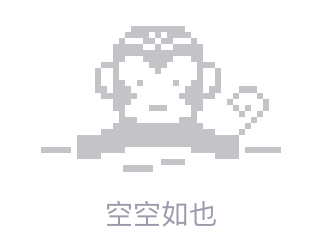# 仅返回一个数据AJAX

Here's what i'm doing. Im trying to return a `data` through my database and i want the `data` to display in an organized manner. I need to distribute the data in different `html tables` based on what `year` or `semester` the data is. If you know about curriculum it's like that.

here's my algorithm. first i initialize a `variable` outside the `while loop` which is `\$a`, if `semester` = `1st` and `year` = `1` then it will go through another if which is checking if `\$a` = 1 if it's equals it will create a `div` that contains part of the `html tables` then it will print out the `data` fetched from the database in `td` after that it will check again if `\$a` = 1 then it will create the last part of the `html tables`.

To summarize. I want to distribute the data based on what semester and year the data is. But the problem is only one data is shown i have two data which equals to `1st semester and year 1`.

``````    \$a = 1;
while(\$row = mysqli_fetch_array(\$result))
{
if(\$row['semester'] == '1st' && \$row['year'] == 1){

/*This if condition below is set to initiliaze the table headers only once*/

if(\$a == 1){
\$output .='<div class="table-responsive">
<table class="table table-striped">
<tr>
<th class="text-center">Subject Code</th>
<th class="text-center">Subject Title</th>
<th class="text-center">Units</th>
<th class="text-center">Pre-req</th>
<th class="text-center">Co-req</th>
</tr>
<tbody>';
}

\$output .= '<tr>
<td class="text-center">'.\$row['code'].'</td>
<td class="text-center">'.\$row['title'].'</td>
<td class="text-center">'.\$row['unit'].'</td>
<td class="text-center">'.\$row['prereq'].'</td>
<td class="text-center">'.\$row['corequisite'].'</td>
</tr>';

/*This if condition below is set to initiliaze the table headers only once*/

if(\$a == 1){
\$output .= '</tbody>
</table>
</div';
\$a = 2;
}

}

}
echo \$output;
``````2018/01/12 15:53
• php
• html
• ajax
• jquery
• 点赞
• 收藏
• 回答
私信

#### 3个回复